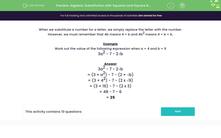# Algebra: Substitution with Squares and Square Roots (2)

In this worksheet, students substitute numbers into expressions containing squares and square roots.Key stage:  KS 3

Curriculum topic:   Algebra

Curriculum subtopic:   Substitute Numerical Values for Formulae/Expressions

Difficulty level:#### Worksheet Overview

When we substitute a number for a letter, we simply replace the letter with the number.

However, we must remember that 4b means 4 × b and 4b2 means 4 × b × b.

Example

Work out the value of the following expression when a = 4 and b = 9

3a2 - 7 - 2√b

3a2 - 7 - 2b

= (3 × a2) - 7 - (2 × b)

= (3 × 42) - 7 - (2 x √9)

= (3 × 16) - 7 - (2 x 3)

= 48 - 7 - 6

= 35

### What is EdPlace?

We're your National Curriculum aligned online education content provider helping each child succeed in English, maths and science from year 1 to GCSE. With an EdPlace account you’ll be able to track and measure progress, helping each child achieve their best. We build confidence and attainment by personalising each child’s learning at a level that suits them.

Get started